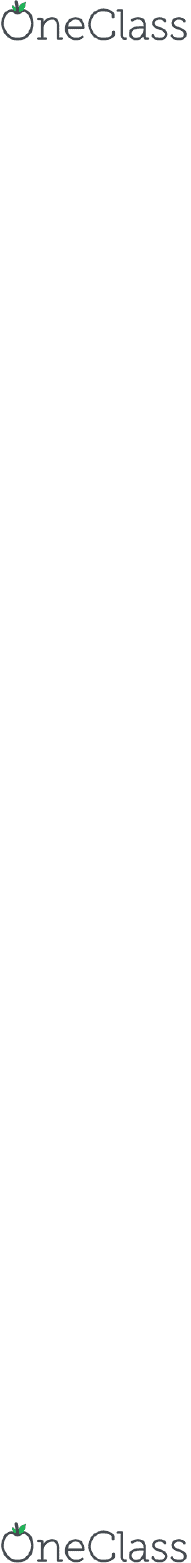Study Guides (380,000)
US (220,000)
UCSB (3,000)
CMPSC (30)
Wang (5)
Study Guide

# CMPSC 40- Midterm Exam Guide - Comprehensive Notes for the exam ( 22 pages long!)

Department
Computer Science
Course Code
CMPSC 40
Professor
Wang
Study Guide
Midterm

This preview shows pages 1-3. to view the full 22 pages of the document.UCSB
CMPSC 40
MIDTERM EXAM
STUDY GUIDE

Only pages 1-3 are available for preview. Some parts have been intentionally blurred.Only pages 1-3 are available for preview. Some parts have been intentionally blurred.History of computing = history of ideas
Aka discrete mathematics
Computer science is built upon ideas in logic, set theory, number theory,
computability, etc.
Thinking and hacking
What is computer science?
Discrete objects and their concepts, properties, relationships
Provable vs assumed
What can we know for certain?
What is unknowable or impractical?
i.e. minimum/maximum/average number of steps to solve a problem
Can we determine important boundaries?
How powerful/thorough/efficient is a particular representation scheme or programming
language?
What common methods can be used to solve a wide variety of real-world computing
problems?
Discrete mathematics
Logical representation and inference
Logical thinking
Sets, orderings, functions, relations, graphs, etc.
Relational thinking
Recursive relations, definitions and structures, induction
Recursive thinking
Counting, arrangements, probability, estimation
Quantitative thinking
Algorithms, complexity, verification
Analytical thinking
Computational thinking includes:
Propositional logic and its applications
Predicate logic
Rules of inference
Proof methods and strategies
Logic
Set composition, operations, and functions
Sequences and summation
Cardinality
Set theory
Algorithms and their properties
The growth of functions, Big-O notation
Algorithm complexity
Algorithms
Integer arithmetic and algorithms
Primes and greatest common divisors
Number theory
Mathematical induction
Strong induction and well ordering
Induction and recursion
Primary course topics
Lecture 1: Propositional Logic
Monday, October 2, 2017
2:00 PM
CMPSC 40 Page 1
find more resources at oneclass.com
find more resources at oneclass.com
###### You're Reading a Preview

Unlock to view full version

Only pages 1-3 are available for preview. Some parts have been intentionally blurred.Strong induction and well ordering
Recursive definitions and algorithms
The basics of counting
The pigeonhole principle
Permutations and combinations
Binomial coefficients and identities
Generalized permutations and combinations
Counting
Important to have rigorous methods to check that programs/systems behave as
expected
Time/space complexity
Important to have methods to analyze the complexity of programs
Primary goal of course is to learn how to construct/read mathematical proofs
Spectrum of formality in proofs
Proof = a derivation that proceeds form a set of hypotheses (premises, axioms) in order to
derive a conclusion, using a set of logical rules
Proofs
Axioms/postulates/hypotheses
Start with basic assumptions (propositions that are undeniably true)
Proof = sequence of logical deductions from axioms and previously-proved statements that
concludes with the proposition in question
Axiomatic method = the standard procedure for establishing truth in mathematics
Propositional logic provides a good foundation for representing and reasoning about facts
Informal language vs logical language
Allowable symbols
Rules for constructing grammatically correct sentences
Just symbols and symbol manipulation
No high-level meaning
Syntax defines the proper sentences in the language
Correspondence (isomorphism) between sentences and facts in the world
Gives an interpretation to the sentence
Sentences can be true or false with respect to the semantics
Semantics defines the meaning of sentences
Syntax and semantics
In contrast to a command, a question, or an exclamation
A sentence in the form of a statement
A sentence that states a fact
Declarative sentence is:
Proposition = declarative sentence that is either true or false
Propositions
Proposition = declarative sentence that is either true or false
Aka postulate, hypothesis
Axiom = proposition that is undeniably (or assumed to be) true
Aka identity, rule, law, principle
Theorem = an important true (proven) proposition
Lemma = a preliminary proposition useful for proving later propositions, typically with little
use otherwise
Corollary = a proposition that follows in just a few logical steps from a theorem
Note different usage of "hypothesis"
Aka hypothesis
Conjecture = an unproven statement that is believed to be true
Terminology
Propositional logic
CMPSC 40 Page 2
find more resources at oneclass.com
find more resources at oneclass.com
###### You're Reading a Preview

Unlock to view full version